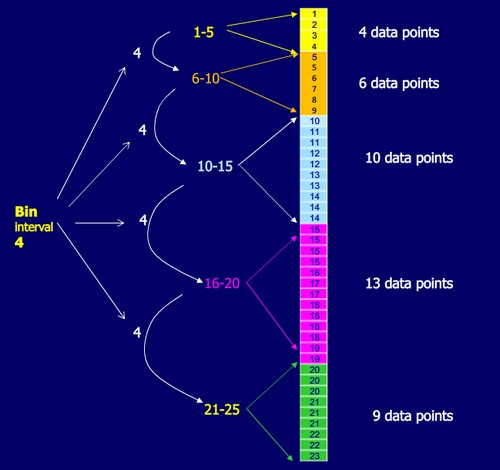# Machine Learning - (Univariate|Simple) Logistic regression

A Simple Logistic regression is a Logistic regression with only one parameters. For the generalization (ie with more than one parameter), see Statistics Learning - Multi-variant logistic regression

Logistic regression comes from the fact that linear regression can also be used to perform classification problem but the logistic regression is not linear (because it involves a transformation with both an exponential function of x and a ratio. See logit_transform)

Just by transforming the categorical target with continuous values.

The idea of logistic regression is to make linear regression produce probabilities. It's always best to predict class probabilities instead of predicting classes.

Logistic regression estimate class probabilities directly using the logit_transform.

The Linear regression calculate a linear function and then a threshold in order to classify.

The result is logistic regression, a popular classification technique.

## Linear regression output as probabilities

It's tempting to use the linear regression output as probabilities but it's a mistake because the output can be negative, and greater than 1 whereas probability can not.

As regression might actually produce probabilities that could be less than 0, or even bigger than 1, logistic regression was introduced.

## The model

### Logit transform

In linear regression, we have a linear sum. In logistic regression, this linear sum are embedded in a formula: the “logit transform”. It's a sort of S-shaped curve that applies a softer function. It's a soft function of a step function (Never below 0, never above 1 and a smooth transition in between).

$$\begin{array}{rrrl} Pr(Y = 1|X) & = & p(X) & = & \frace^B_0 + B_1 . X}}1+ e^B_0 + B_1 . X}} \\ \end{array}$$

where:

• $e \approx 2:71828$ is the scientific constant, the exponential. Euler's number

The values have to lie between 0 and 1 because:

• e to anything is positive.
• As the denominator is bigger than the numerator, it's always got to be bigger than 0.
• When $B_0 + B_1 X$ gets very large, this approaches 1.

So this is a special construct, a transformation of a linear model to guarantee that what we get out is a probability.

With a bit of rearrangement: $$\begin{array}{rrl} log \left (\frac p(X)}1 - p(X)} \right ) & = & B_0 + B_1 X \\ \end{array}$$

The name “logistic” comes from the transformation of this model. This is a monotone transformation. And that transformation is called:

• the log odds
• or the logit transformation of the probability.

To summarize, we got still a linear model but it's modeling the probabilities on a non-linear scale.

### Parameters Estimation

With the model above, how do we estimate the parameters from the data?

The popular way is to use maximum likelihood:

### Interpretation

The P-value for the intercept is not really important. The intercept has largely to do with the preponderance of 0's and 1's in the data set. The slope is really important.

## Note

### Probability of 50%

You get a probability of 50%, when

$$\begin{array}{rrl} e^B_0+B_1.X} & = & 1 \\ X & = & \frac{-B_0}{B_1} \end{array}$$

## Weka

Function > Logistic

Recommended PagesData Mining - Maximum Entropy Algorithm

Maximum Entropy (MaxEnt) models are feature-based classifier models. In a two-class scenario, it is the same as using logistic regression to find a distribution over the classes. MaxEnt makes no...Machine Learning - Logistic regression (Classification Algorithm)

The prediction from a logistic regression model can be interpreted as the probability that the label is 1. linear regression can also be used to perform classification problem. Just by transforming the...Statistics - (Discretizing|binning) (bin)

Discretization is the process of transforming numeric variables into nominal variables called bin. The created variables are nominal but are ordered (which is a concept that you will not find in true...